# Derivative of a quadratic form

Jump to: navigation, search

Let$A \in \mathcal M_{n,n}(\mathbf R)$ be an$n$ by$n$ symmetric real-valued matrix, and let$f\colon \mathbf R^n \to \mathbf R$ be defined by$f(x) = x^{\mathrm T}Ax$. On this page, we calculate the derivative of$f$ using three methods.

## Understanding the problem

Since$f$ is a real-valued function of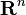$\mathbf R^n$, the derivative and the gradient coincide.

## Straightforward method

This method is the most straightforward, and involves breaking apart the matrix and vector into components and performing the differentiation. While straightforward, it appears messy due to the indices involved.

Let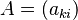$A = (a_{ki})$ and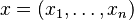$x = (x_1,\ldots,x_n)$.

We expand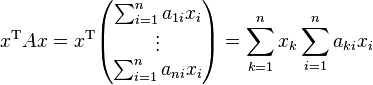$x^{\mathrm T}Ax = x^{\mathrm T}\begin{pmatrix}\sum_{i=1}^n a_{1i}x_i \\ \vdots \\ \sum_{i=1}^n a_{ni}x_i\end{pmatrix} = \sum_{k=1}^n x_k \sum_{i=1}^n a_{ki}x_i$

Now we find the partial derivative of the above with respect to$x_j$. To distinguish the constants from the variable, it makes sense to split the sum: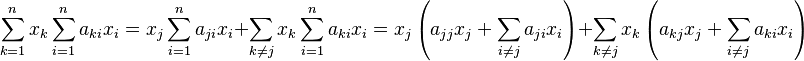$\sum_{k=1}^n x_k \sum_{i=1}^n a_{ki}x_i = x_j \sum_{i=1}^n a_{ji}x_i + \sum_{k\ne j} x_k \sum_{i=1}^n a_{ki}x_i = x_j\left(a_{jj}x_j + \sum_{i\ne j} a_{ji} x_i\right) + \sum_{k\ne j} x_k \left(a_{kj}x_j + \sum_{i\ne j} a_{ki} x_i\right)$

The first equality comes from splitting the outer summation, and the second comes from splitting the two inner summations.

Now distributing we have\begin{align}a_{jj}x_j^2 + \left(\sum_{i\ne j} a_{ji} x_i\right)x_j + \sum_{k\ne j} \left(a_{kj}x_k x_j + x_k \sum_{i\ne j} a_{ki} x_i\right) \\ = a_{jj}x_j^2 + \left(\sum_{i\ne j} a_{ji} x_i\right)x_j + \left(\sum_{k\ne j}a_{kj}x_k\right) x_j + \sum_{k\ne j}x_k \sum_{i\ne j} a_{ki} x_i\end{align}

It is now easy to do the differentiation. We obtain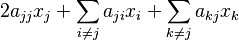$2a_{jj}x_j + \sum_{i\ne j} a_{ji} x_i + \sum_{k\ne j}a_{kj}x_k$

Since the matrix is symmetric,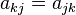$a_{kj} = a_{jk}$ so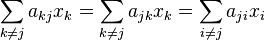$\sum_{k\ne j}a_{kj}x_k = \sum_{k\ne j}a_{jk}x_k = \sum_{i\ne j}a_{ji}x_i$. The final equality follows because$k$ is just an indexing variable and we are free to rename it. But now the derivative becomes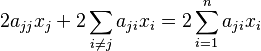$2a_{jj}x_j + 2\sum_{i\ne j} a_{ji} x_i = 2\sum_{i=1}^n a_{ji} x_i$

But this is just the$j$th component of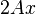$2Ax$. It follows that the full derivative is just$2Ax$ (or its transpose, depending on whether we want to view it as a row or column vector).

## Using the definition of the derivative

This is an expanded version of the answer at .

Using the definition, we can compute the derivative from first principles without exposing the components.

The derivative is the linear transformation$L$ such that: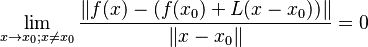$\lim_{x\to x_0; x\ne x_0} \frac{\|f(x) - (f(x_0) + L(x-x_0))\|}{\|x-x_0\|} = 0$

Using our function, this is:$\lim_{x\to x_0; x\ne x_0} \frac{\|x^{\mathrm T}Ax - x_0^{\mathrm T}Ax_0 - L(x-x_0)\|}{\|x-x_0\|} = 0$

Defining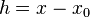$h = x-x_0$, we have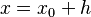$x = x_0 + h$ and$\frac{\|(x_0 + h)^{\mathrm T}A(x_0 + h) - x_0^{\mathrm T}Ax_0 - L(h)\|}{\|h\|}$

Focusing on the subexpression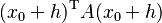$(x_0 + h)^{\mathrm T}A(x_0 + h)$, since$A$ is a matrix, it is a linear transformation, so we obtain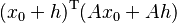$(x_0 + h)^{\mathrm T}(Ax_0 + Ah)$. Since the transpose of a sum is the sum of the transposes, we have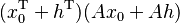$(x_0^{\mathrm T} + h^{\mathrm T})(Ax_0 + Ah)$. Now using linearity we have$x_0^{\mathrm T}Ax_0 + h^{\mathrm T} Ax_0 + x_0^{\mathrm T} Ah + h^{\mathrm T}Ah$.

Now the fraction is$\frac{\|x_0^{\mathrm T}Ax_0 + h^{\mathrm T} Ax_0 + x_0^{\mathrm T} Ah + h^{\mathrm T}Ah - x_0^{\mathrm T}Ax_0 - L(h)\|}{\|h\|} = \frac{\|h^{\mathrm T} Ax_0 + x_0^{\mathrm T} Ah + h^{\mathrm T}Ah - L(h)\|}{\|h\|}$

Focusing on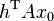$h^{\mathrm T} Ax_0$, it is a real number so taking the transpose leaves it unchanged: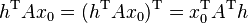$h^{\mathrm T} Ax_0 = (h^{\mathrm T} Ax_0)^{\mathrm T} = x_0^{\mathrm T}A^{\mathrm T}h$.

Now the fraction is$\frac{\|x_0^{\mathrm T}A^{\mathrm T}h + x_0^{\mathrm T} Ah + h^{\mathrm T}Ah - L(h)\|}{\|h\|} = \frac{\|x_0^{\mathrm T}(A^{\mathrm T} + A)h + h^{\mathrm T}Ah - L(h)\|}{\|h\|}$

In the numerator,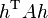$h^{\mathrm T}Ah$ is a higher order term that will disappear when taking the limit, so the linear transformation we are looking for must be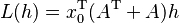$L(h) = x_0^{\mathrm T}(A^{\mathrm T} + A)h$. Since$A$ is symmetric, we have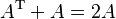$A^{\mathrm T} + A = 2A$ and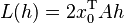$L(h) = 2x_0^{\mathrm T}Ah$.

## Using the chain rule

In this approach, we think of$f$ as a composition of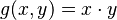$g(x,y) = x\cdot y$ and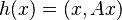$h(x) = (x, Ax)$ and use the multivariable chain rule.

Define:

•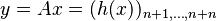$y = Ax = (h(x))_{n+1,\ldots,n+n}$
•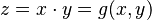$z = x\cdot y = g(x,y)$

What is tricky is that$y$ is not$h(x)$; to make the composition work, we must stick on$x$ to$y$ to form$(x,y)$ before passing to$g$.

Now the multivariable chain rule says:$\frac{\partial z}{\partial x_j} = \underbrace{\frac{\partial z}{\partial x_1}\frac{\partial x_1}{\partial x_j} + \cdots + \frac{\partial z}{\partial x_n}\frac{\partial x_n}{\partial x_j}}_{\text{first half of terms}} + \underbrace{\frac{\partial z}{\partial y_1}\frac{\partial y_1}{\partial x_j} + \cdots + \frac{\partial z}{\partial y_n}\frac{\partial y_n}{\partial x_j}}_{\text{second half of terms}}$

The notation is confusing because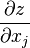$\frac{\partial z}{\partial x_j}$ means different things on each side of the equation (since$x$ is both the input variable and an intermediate variable).

Looking only at the first half of the terms,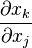$\frac{\partial x_k}{\partial x_j}$ is$1$ if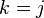$k=j$ and$0$ otherwise, so we keep only the$j$th term, where we see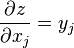$\frac{\partial z}{\partial x_j} = y_j$.

Now looking at the second half of the terms,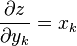$\frac{\partial z}{\partial y_k} = x_k$ and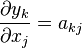$\frac{\partial y_k}{\partial x_j} = a_{kj}$.

Putting all the above together, we obtain$\frac{\partial z}{\partial x_j} = y_j + x_1 a_{1j} + \cdots + x_n a_{nj} = 2y_j$

In the last equality we used the fact that$A$ is symmetric.

We now have the$j$th component of the derivative, so the full derivative is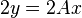$2y = 2Ax$.

See  for something similar.